# Test: Exponents- 2

## 20 Questions MCQ Test General Aptitude for GATE | Test: Exponents- 2

Description
Attempt Test: Exponents- 2 | 20 questions in 40 minutes | Mock test for Quant preparation | Free important questions MCQ to study General Aptitude for GATE for Quant Exam | Download free PDF with solutions
QUESTION: 1

### If Z is a positive integer such that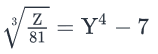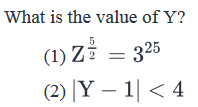Solution:

Steps 1 & 2: Understand Question and Draw Inferences

Given:

• Z is a positive integer
• Z = 81(Y4 – 7)3  . . . (1)

We need to find the value of Y.

Step 3: Analyze Statement 1 independently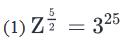• Squaring both sides:
• Z5 = 350
• Taking 5th root on both sides:
• Z = 310 . . . (2)
• Put (2) in (1):
• 310 = 34(Y4 – 7)3
• 36 = (Y4 – 7)3
• Taking the cube-root on both sides:
• 32 = Y4 – 7
•  Y4 = 9 + 7 = 16
• Y4 = 24 = (-2)4
• Y = 2 or -2

Not sufficient to determine a unique value of Y.

Step 4: Analyze Statement 2 independently

(2) |Y-1| < 4

• Distance of Y from 1 on the number line is less than 4 units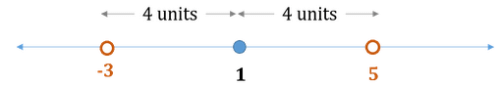• -3 < Y < 5

Multiple values of Y possible. Not sufficient.

Step 5: Analyze Both Statements Together (if needed)

• From St. 1, Y = 2 or – 2
• From St. 2, -3 < Y < 5
• This inequality is satisfied by both 2 and -2

So, even after combining both statements, we have 2 possible values of Y

Since we couldn’t find a unique value of Y, the correct answer. Is Option E.

QUESTION: 2

### Simplify the given expression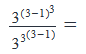Solution: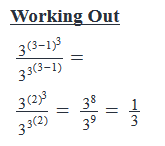QUESTION: 3

### What is the value of x if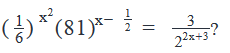Solution:

Working Out:• Since x is in the powers of different bases in this equation, to get equations in x, we need to be able to equate the bases on the two sides of the equation
• Expressing each base in terms of its prime factors: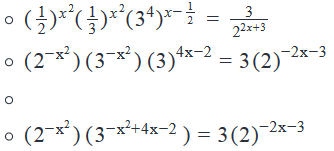Equating the powers of base 2 on both sides: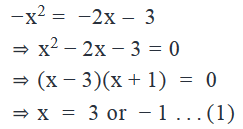Equating the powers of base 3 on both sides: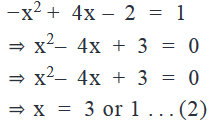The value of x that satisfies both (1) and (2) is:

x = 3

QUESTION: 4

If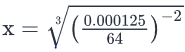, what is the value of x?

Solution:

Given:To find: Value of x

Working Out: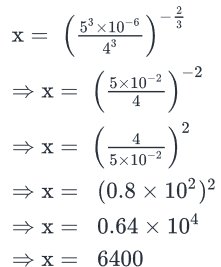Looking at the answer choices, we see that the correct answer is Option A

QUESTION: 5

If x and y are non-zero numbers, what is the value of y?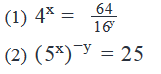Solution:

Steps 1 & 2: Understand Question and Draw Inferences

Given: x ≠ 0, y ≠ 0

To find:  y = ?

Step 3: Analyze Statement 1 independently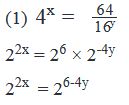Equating the powers of 2 on both sides:

2x = 6 – 4y

x + 2y = 3

1 Linear Equation with 2 unknowns. Not sufficient to find a unique value of y.

Step 4: Analyze Statement 2 independently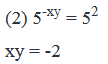Not sufficient to find a unique value of y.

Step 5: Analyze Both Statements Together (if needed)

• From Statement 1: x + 2y = 3 . . . (1)
• From Statement 2: xy = -2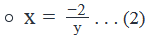Substituting (2) in (1):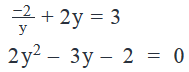• This quadratic equation gives 2 values of y
• The only constraint on y: y ≠ 0
• Since 0 is not a root of the above quadratic equation, this constraint doesn’t help eliminate one of the two roots of y

• Thus, 2 values of y are obtained from the combination of the two statements

Not sufficient to obtain a unique value of y.

QUESTION: 6

If x > 0 and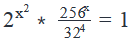, what is the value of x?

Solution:

Given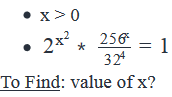Approach

1. We can see that the terms on the left hand side of the equation are powers of 2.
1. So, we will simplify the expression in powers of 2.
2. So, we will get 2expression in x = 1. This is only possible if expression in x = 0 (as 2 = 1)
3. We will equate the expression in x = 0 and use the constraint that x > 0, to find out the value of x.

Working Out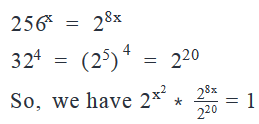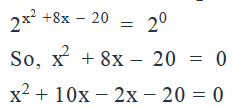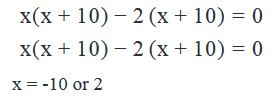As x> 0, the only possible value of x = 2.

QUESTION: 7

If x and y are positive integers, what is the remainder when y is divided by x?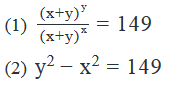Solution:

Steps 1 & 2: Understand Question and Draw Inferences

• Given: x, y are integers > 0
• y = ax + r , where a is a positive integer and r is an integer such that 0 ≤ r < x

To Find: Value of r for which we need to find the value of x and y.

Step 3: Analyze Statement 1 independently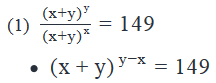• As 149 is expressed as an integer raised to a power, we need to find that integer. As this integer will be a factor of 149, we need to find if 149 can be expressed as a factor raised to its power. So, let’s check its divisibility by the prime numbers.
• Divisibility by 2: As 149 is odd, it is not divisible by 2
• Divisibility by 3: As the sum of the digits of 149 (i.e. 14) is not divisible by 3, 149 will not be divisible by 3
• Divisibility by 5: As the units digit of 149 is neither 0 nor 5, it is not divisible by 5
• Divisibility by 7: We can see that 149 when divided by 7, leaves a remainder 2. So, it is not divisible by 7
• Divisibility by 11: 149 when divided by 11 leaves a remainder 4. So, it is not divisible by 11
• Divisibility by 13: We see that 149 when divided by 13 leaves a remainder 6. So, it is not divisible by 13.
• Since 122 < 149 < 132 and 149 is not divisible by any prime number till 13, we can say that 149 is a prime number.
• As 149 is a prime number, any integer apart from 149 raised to any power will not result in 149.
• Only possible option is x + y = 149 and y – x = 1
• 2 equations, 2 variables, we can find unique values of x and y and hence find the remainder.

Step 4: Analyze Statement 2 independently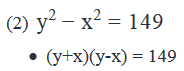• So, we need to express 149 as a product of 2 integers. For expressing 149 as a product of 2 integers, we need to find the factors of 149.
• As we have found above that 149 is a prime number, the only possible case of expressing 149 as a product of 2 integers is 149 * 1
• y + x = 149 and y – x = 1
• 2 equations, 2 variables, we can find unique values of x and y and hence find the remainder.

Step 5: Analyze Both Statements Together (if needed)

As we have a unique answer from steps 3 and 4, this step is not required.

QUESTION: 8

If a, b and x are integers such that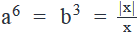, what is the value of a - b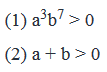Solution:

Steps 1 & 2: Understand Question and Draw Inferences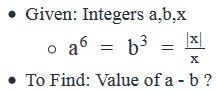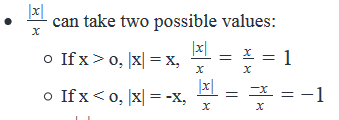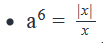• As a6 is always positive,a= 1, i.e. a = 1 or -1
• So, we can reject the value of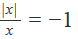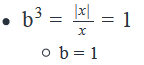Possible values of a – b

• If a = 1 and b = 1, a – b = 0
• If a = -1 and b = 1, a- b = -2

• So, we need to find the unique value of a to find the value of a – b.

Step 3: Analyze Statement 1 independently

(1) a3 b7 > 0

• Rewriting a3b7 as ab(a2b6)
• Therefore, ab(a2b6)>0
• We know that a2b6 is always > 0 (even power of any number is always positive)
• So, for ab(a2b6)> 0
•   ab > 0
• This tells us that a and b have same signs.
• Since b > 0, therefore a will also be greater than 0, so the value of a = 1.
• a – b = 1 -1 = 0

Step 4: Analyze Statement 2 independently

(2) a + b > 0

• If a = 1 and b = 1, a + b = 2 > 0
• If a = -1 and b = 1, a + b = 0, is not greater than zero
• Hence, we have a unique answer, where a =1 and b = 1

Thus a – b = 1 – 1 = 0.

Step 5: Analyze Both Statements Together (if needed)

As we have a unique answer from steps 3 and 4, this step is not required.

QUESTION: 9

A function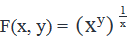for positive integers x and y. Is F(a, b) > a, where a and b are positive integers?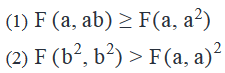Solution:

Steps 1 & 2: Understand Question and Draw Inferences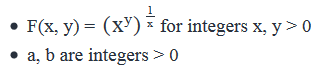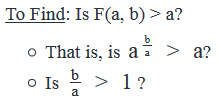• Since a is a positive integer, multiplying both sides of the inequality doesn’t impact the sign of inequality
• So, the question simplifies to: is b > a?

Step 3: Analyze Statement 1 independently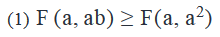Using the definition of this function, we can write: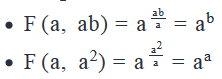So as per Statement 1: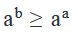As a is a positive integer,aa > 0. So, we can divide both sides of the inequality by aa without changing the sign of the inequality.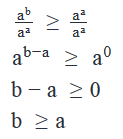So, b > a or b = a.

Hence, we can not say for sure if b > a. Insufficient to answer.

Step 4: Analyze Statement 2 independently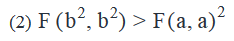Using the definition of this function, we can write: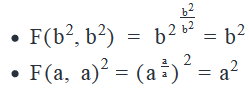So, as per statement-2,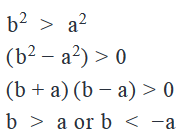• As a > 0, - a < 0. So b < -a is not possible as it will mean that b is negative.
• So, the only possible case is  b > a

Step 5: Analyze Both Statements Together (if needed)

As we have a unique answer from step – 4, this step is not required.

QUESTION: 10

Is the sum of xy and yx positive?

(1) xy > 0

(2) x + y > 0

Solution:

Steps 1 & 2: Understand Question and Draw Inferences

To Find: If xy + yx > 0

Step 3: Analyze Statement 1 independently

(1) xy > 0

• Tells that x and y are of the same sign. Two cases arise:
• x, y > 0
• In this case xy, yx > 0. So, xy + yx > 0
• x, y < 0
• In this case, xy + yx may or may not be positive, depending on the values of x and y. Following cases can arise:
• Both x, y are even → In this case xy,yx  > 0. So, xy+yx > 0
• Both x and y are odd → In this case xy,yx < 0. So, xy+yx < 0
• x is even and y is odd → In this case xy < 0, yx > 0. Cannot comment on the value of xy+yx
• x is odd and y is even → In this case xy < 0, yx > 0. Cannot comment on the value of xy+yx

Step 4: Analyze Statement 2 independently

(2) x + y > 0

• Considering the constraint x + y > 0, following cases are possible:
• x, y > 0. So, x + y > 0. In this case, xy, yx > 0. So, xy + yx > 0
• x < 0, y > 0 and |y| > |x|. So, x + y > 0. In this case xy + yx may or may not be positive.
• If y is odd, then xy < 0 , yx > 0 as y is positive. In this case, we cannot comment on the value of xy + yx
• If y is even , then xy > 0 , yx > 0 as y is positive. In this case, xy + yx > 0
• x > 0, y < 0 and |x| > |y|. So, x + y > 0. In this case xy + yx may or may not be positive.

Step 5: Analyze Both Statements Together (if needed)

1. From Statement 1, xy > 0
2. From Statement 2, x + y > 0

Statement-1 tells us that x and y have the same signs. Following cases are possible:

• If x & y > 0, xy > 0 and x + y > 0. In this case xy + yx > 0
• If x & y < 0, x+ y < 0. Not possible. (If x & y both are negative, the x + y cannot be > 0)

The only possible case is when x, y > 0 and hence xy + yx > 0

QUESTION: 11

What is the remainder obtained when 1010 + 105 – 24 is divided by 36?

Solution:

Given:

• Not applicable

To find: The remainder when 1010 + 105 – 24 is divided by 36

Approach:

1. Let the required remainder be r. This means, we will be able to write:
1010 + 105 – 24 = 36k + r, where quotient k is an integer and 0 ≤ r < 36
The above expression is our GOAL expression. We’ll try to simplify the dividend 1010 + 105 – 24 till it is comparable to our GOAL expression, and then, by comparison, we’ll be able to find the value of r.

Working Out:

• 1010 + 105 – 24 = 1005 + 1002*10 – (36 – 12)
• =(36*3 – 8)5 + (36*3 – 8)2*10 – 36 + 12
• Now, from Binomial Theorem, we know that every term in the expansion of (36*3 – 8)5 will be divisible by 36, except the last term, and the last term will be (-8)5
• So, we can write: (36*3 – 8)5 is of the form 36a + (-8)5, where 36a is a catch-all term conveying that all the other terms in this expansion are divisible by 36
• Similarly, every term in the expansion of (36*3 – 8)2 will be divisible by 36 except the last term, and the last term will be (-8)2
• So, we can write: (36*3 – 8)2 = 36b + (-8)2
• So, the given expression simplifies to:
• {36a + (-8)5 } + {36b + (-8)2}*10 – 36 + 12
• = (36a + 360b – 36) + (-85 + 640 + 12)
• = (36a + 360b – 36) + (-85+ 652)
• = (36a + 360b – 36 + 648) + (-85+ 4)
• = (36a + 360b – 36 + 36*18) + (-85+ 4)
• The above expression is not comparable to our GOAL expression because the term -85 in it is still unresolved. Do we need to calculate the value of -85 to answer this question? No. We only need to express it in terms of 36. Once again, we’ll use Binomial Theorem to do so:
• -85 = -8(82)2 = -8(64)2 = -8(36*2 – 8)2
• Every term in the expansion of (36*2 – 8)2 will be divisible by 36 except the last term. The last term will be (-8)2 = 64
• So, the expression (36*2 – 8)2 can be written as: 36c + 64
• So, -8(36*2 – 8)2 = -8(36c + 64)
• =-8(36c + 36*2 – 8)
• = (-8*36c – 8*36*2) + 64
• So, the given expression simplifies to: (36a + 360b – 36 + 72) + {(-8*36c – 8*36*2) + 64} + 4
• = (36a + 360b – 36 + 72 – 8*36c – 8*36*2) + 68
• =(36a + 360b – 36 + 72 – 8*36c – 8*36*2 + 36) + 32
• Now, the above expression is exactly comparable to our GOAL Expression: 36k + r
• So, by comparison, we can say that Remainder r = 32

Looking at the answer choices, we see that the correct answer is Option E

QUESTION: 12

The distance between celestial bodies is expressed in terms of light years, where 1 light year is the distance travelled by light in one year and is equal to 9.46 X 1017 centimetres. If light takes 499 seconds to travel from the Sun to the Earth, which of the following is the closest to the distance, in million kilometres, between the Earth and the Sun?

Solution:

Given:

• 1 light year = Distance travelled by light in 1 year = 9.46 x 1017 centimeters
• Time taken by light to travel from Sun to Earth = 499 seconds

To find: The option that is closest to the distance, in million kilometers, between Sun and Earth

Approach:

1. To answer the question, we need to find the approximate distance between Sun and Earth
• Note that the 5 answer choices are quite far apart in magnitude. Therefore, we’ll be able to use Estimation and Rounding aggressively. All we need here is an idea of the order of the magnitude of the distance between Sun and Earth (whether this distance is in hundred millions or thousand millions or ten thousand millions of kiometers).
2. From the definition of light year, we get the distance travelled by light in 1 year. So, using the Unitary Method, we’ll be able to find the distance travelled by light in 499 seconds (and thus, the distance between Sun and Earth)
• One thing that we must be careful about when working out this solution – we must use one unit of time (either years or seconds) and one unit of distance (either centimeters or kilometers) in our solution.
• Working Out:

• Expressing the given information in a single Distance unit and a single Time unit
• Since the answer choices are in units of (millions of) kilometers, it’ll be wise for us to convert the distance given in centimeters into kilometers. (If you want to do vice-versa, that is, if you want to convert kilometers into centimeters, then you may later have to do 5 such conversions – 1 for each answer choice and therefore, that will be more time-consuming)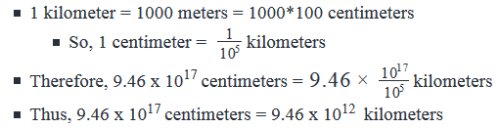• For Time, let’s convert 1 year into seconds:
• 1 year = 365*24*60*60 seconds
• = 36*24*36*103 seconds approx.
• (Note: we’re not simplifying this expression very much here because we’ll do all the simplifications together after we’ve deduced an expression for the distance between Earth and Sun)
• Finding the approximate distance between Earth and Sun
• From the definition of light year, we know that: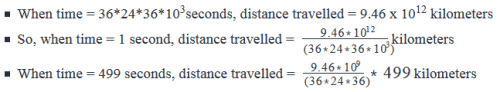• Thus, distance between Earth and Sun =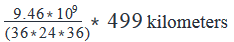• We’ll now simplify the above expression for distance by using estimation and rounding: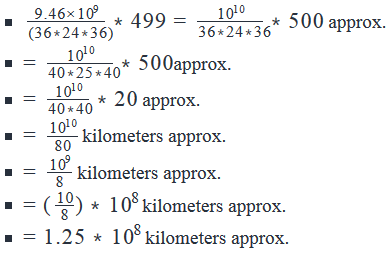• Since the question asks for the distance in units of million kilometers, let’s now express the above distance in million kilometers: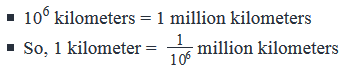Therefore, 1.25*108 kilometers =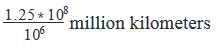• = 1.25*102 million kilometers
• = 125 million kilometers approx.
• Thus, we see that the order of distance between the Sun and the Earth is hundred million kilometers.

Looking at the answer choices, we see that the correct answer is Option A

QUESTION: 13

James deposited \$1,000 each in two investment schemes X and Y. Scheme X doubles the invested amount  every 7 years and scheme Y doubles the invested amount every 14 years. If James withdraws \$500 from scheme X at the end of every 7th year, how many years will it take for the total amount invested in schemes X and Y to amount more than \$40,000?

Solution:

Given

• Scheme X doubles the invested amount every 7 years
• James deposited \$1000 in scheme X
• James withdraws \$500 from scheme X after the end of every 7 years

• Scheme Y doubles the invested amount after every 14 years
• James deposited \$1,000 in scheme Y

To Find: Number of years it will take total amount deposited in schemes X and Y to grow to > \$40,000?

Approach

1. For finding the number of years it will take the deposits in schemes X and Y to grow to more than \$40,000, we need to find the amount in both the schemes X and Y after every 7 years.(As amount in scheme X doubles after every 7 years, we will need to calculate the amount at the end of every 7 years and not at the end of 14 years).
2. Scheme X
1. As the amount invested in scheme X doubles every 7 years, we will need to calculate the amount in scheme X after every interval of 7 years
2. However, we will need to make sure that we subtract \$500 at each interval of 7 years from the final amount
3. Scheme Y
1. As the amount invested in scheme Y doubles after every 14 years, we will need to calculate the amount in scheme Y after every interval of 14 years.
4. At each interval, we will calculate the sum of amounts in scheme X and Y to check if it exceeds \$40,000.

Working Out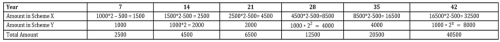1. Amount at the end of year 7 in scheme X = \$1000 * 2 = \$2000
1. However James withdrew \$500 at the end of 7th year, So, the amount remaining will be \$2000 – \$500 = \$1500
2. The same logic has been applied in calculating the amounts at the end of every 7 year interval

2. Amount at the end of year 14 in scheme Y = \$1000 * 2 = \$2000
1. The same logic has been applied in calculating the amounts at the end of every 14 years interval.

3. We can see that the total amount in schemes X and Y exceed \$40,000 by the end of the year 42.

QUESTION: 14

If x is a positive integer less than 100 such that x is divisible by 2y, where y is a positive integer, what is the value of y?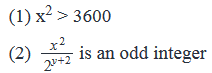Solution:

Steps 1 & 2: Understand Question and Draw Inferences

• x is an integer such that 0 < x < 100
• x is divisible by 2y, where y is a positive integer
• Since y > 0, this means 2 is definitely a prime factor 2
• Since x is a positive integer, we can write the Prime-factorized form of x as: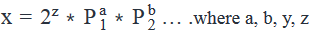• are integers > 0, z ≥ y and   are prime numbers other than 2.
• As x < 100, 2z < 100
• So, z = { 1, 2, 3, 4, 5, 6} as 27 = 128 > 100
• Since x is completely divisible by 2y, z ≥ y. So, y can take any value of z, i.e. 1 ≤ y ≤ 6
• To Find: Unique value of y

Step 3: Analyze Statement 1 independently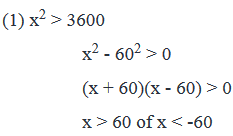• As x is a positive integer, x < -60 is not possible.
• So, x > 60, i.e. 60 < x < 100.

However, we do not know if 2 is the only prime factor or x. So, we cannot find a unique value of y.

• For example, if 2 is the only prime factor of x, then y can have only 1 value: 6
• But if x has other prime factors, then multiple values of y are possible. For example, x could be 22*3*7 (y = 2) or 23*11 (y = 3) etc.

Step 4: Analyze Statement 2 independently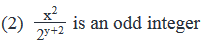• Using the prime factorized expression of x, we can write: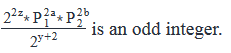• Since the integer resulting from this division is odd, the powers of 2 in the numerator and the denominator should cancel out each other.
• Hence 22z = 2y+2
• z=y/2+1 ….(1). So, y must be even as z is an integer
• Also, from our discussion in Steps 1 and 2, we know that z ≥ y
• Substituting (1) in the above inequality, we get: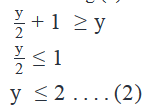Using (2), along with the inference that y must be even, we have y = 2 as the only possible option.

Step 5: Analyze Both Statements Together (if needed)

As we have a unique answer from step 4, this step is not required.

QUESTION: 15

Does ab lie between 0 and 1, exclusive?

(1) |a| < 1

(2) b is a positive odd integer

Solution:

Steps 1 & 2: Understand Question and Draw Inferences

Given: Two numbers a and b

To Find: Is 0 < ab < 1

Following cases are possible:

1. a ≥ 1 →
• If b≥  0, then a ≥ 1. So, the answer to the question is NO
• If b < 0, then 0 < a< 1. So, the answer to the question is YES

2. 0 < a < 1 → Irrespective of the value of b, 0 < ab < 1 So, the answer to the question is YES

3. If a = 0 → Irrespective of the value of b, ab = 0. So, the answer to the question is NO.

4. -1 < a < 0 →

• If b is even, 0 < ab < 1 So, the answer to the question is YES.
• If b is odd, -1 < ab < 0. So, the answer to the question is NO.

5. a ≤ -1 →

• If b is even,ab≥1. So, the answer to the question is NO.
• If b is odd, ab≤1. So, the answer to the question is NO

So, the answer to the question will be YES, if:

• a ≥  1 and b < 0 OR
• 0 < a < 1 OR
• -1 < a < 0 and b is even

And the answer to the question will be NO, if:

• a ≥  1 and b ≥ 0 OR
• a = 0 OR
• -1 < a < 0 and b is odd OR
• a ≤ -1

So, we can answer the given question with a unique answer for the above cases.

Step 3: Analyze Statement 1 independently

(1) |a| < 1

• This means, -1 <  a  < 1. So, following cases can occur:
• -1 < a < 0 → As we have seen from our analysis above that in this case the answer to the question may be YES or NO
• Now, we don’t need to analyse other cases as we do not have unique from this case itself, but we will do so for the sake of completing the analysis.
• a = 0 → The answer to the question is NO in this case
• 0 <  a < 1 → The answer to the question is YES in this case.
• As we do not have a unique answer , we cannot say for sure if 0 < ab < 1

Step 4: Analyze Statement 2 independently

(2) b is a positive odd integer

• We have seen from our analysis in steps 1&2 that we need to find the range of values of a to answer this question.
• As we do not know the value of a, the statement is insufficient to tell if 0 < ab < 1

Step 5: Analyze Both Statements Together (if needed)

(1)From Statement 1,  -1 <  a  < 1

(2) From Statement 2, b > 0

Following cases are possible:

• -1 < a < 0 → We have seen from our analysis in steps 1&2 that if b is odd, the answer to the question is NO.
• a = 0 → We have seen from our analysis in steps 1&2 that in this case the answer to the question is NO.
• 0 < a < 1 → We have seen from our analysis in steps 1&2 that in this case the answer to the question is YES

As we do not have a unique answer, the combination of statements is insufficient to answer.

QUESTION: 16

Z=2a×5b×7c

A positive integer Z can be expressed in terms of its prime factors as above, where a, b and c are positive integers. Is 3|a−b|<9?

(1) Z is divisible by 40 but not by 50

(2) Za−b=26×52×74

Solution:

Steps 1 & 2: Understand Question and Draw Inferences

Given: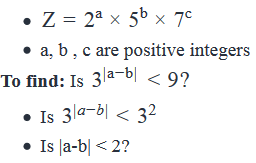Step 3: Analyze Statement 1 independently

(1) Z is divisible by 40 but not by 50

• 40 = 23*5
• 50 = 2*52

• The power of 2 in Z is at least 3
• a ≥ 3
• The power of 5 in Z is 1.
• b = 1

• Therefore, a – b ≥ 3 – 1

a – b ≥ 2

Statement 1 is sufficient to answer the question.

Step 4: Analyze Statement 2 independently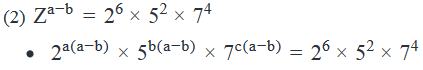• Equating the powers of a base on both sides of the equation:
• a(a - b) = 6. . . (1)
• b(a - b) = 2. . . (2)
• Dividing (1) by (2)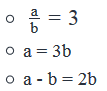• The minimum value of b is 1 (b is a positive integer)
• So, minimum value of a – b = 2

Statement 2 is sufficient to answer the question.

Step 5: Analyze Both Statements Together (if needed)

Since we’ve already arrived at unique answers in Steps 3 and 4, this step is not required

QUESTION: 17

What is the maximum possible power of 4 in the number that is obtained when the product of the first 15 positive integers is subtracted from the product of the first 20 positive integers?

Solution:

Given

• Product of first 15 positive integers = 1*2*3…….* 15 = 15!

• Product of first 20 positive integers = 1*2*3*…….* 20 = 20!
• So, we can write 20! = 15! * 16 * 17 * 18 * 19 * 20

To Find: Maximum power of 4 that divides (20! – 15!)

Approach

1. We will first need to simplify the expression 20! – 15!
1. We know that 20! = 15! * 16 * 17 * 18 * 19 * 20
2. So, 20! – 15! = 15! (20*19*18….*16 – 1) = 15! * odd integer
1. Since 20 *19*…..16 is even, and 1 is odd, 20*19*……16 -1 will be odd
3. Therefore, we only need to find the maximum power of 4 that divides 15!

2. To find the maximum power of 4 in 15!, we will first need to find the maximum power of 2 in 15!, as 4 = 22

1. Let’s take a simple example to understand how we can find this. Consider a number p = 1*2*3*4. We need to find the power of 2 in p.
2. Now, powers of 2 will occur in multiples of 2. So, we should first find the multiples of 2 in p. They are 2 and 4. However all multiples of 2 will not contain only 21. Some of them may contain higher powers of 2. For example, here 4 contains 22.
3. So, when we are finding multiples of 2, we should also find the multiples of higher powers of 2 in p.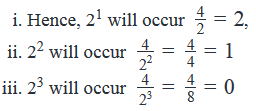4.   Hence, 2 will occur 2 + 1 = 3 times in p.

3. We will use the same logic to find out the power of 2 in 15!

1. Once we know the power of 2 in 15!, we can find the power of 4 in 15!

Working Out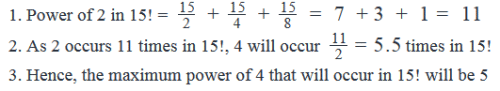QUESTION: 18

For any integer z, the function PODD(z) is defined as the product of all odd integers between –z and z, inclusive. Which of the following numbers will not divide PODD (-15) completely?

I. 38

II. 55

III. 73

Solution:

Given: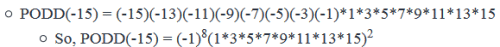To find: PODD(-15) will not be divisible by which of the given 3 options?

Approach:

1. The given 3 options involve powers of 3, 5 and 7. So, to answer the question, we’ll find the powers of 3, 5 and 7 in the prime-factorized expression of PODD(-15)
2. The answer for an option will be YES (meaning that option will not divide PODD(-15) completely) if the power of the prime factor in that option is greater than the power of that prime factor in the prime-factorized expression for PODD(-15)

(For example, 25 will not divide a number of the form 24*3)

Working Out:

• Finding the power of 3 in PODD(-15) and evaluating Option I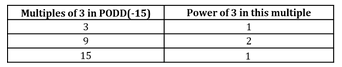• Look again at the expression for PODD(-15):
• PODD(-15) = (-1)8(1*3*5*7*9*11*13*15)2
• Note that each of the above multiples of 3 occurs twice in PODD(-15)
• So, power of 3 in PODD(-15) = (1+2+1)*2= 4*2 = 8
• Since the power of 3 in Option I is equal to the power of 3 in PODD(-15), 38 will divide PODD(-15) completely.

Finding the power of 5 in PODD(-15) and evaluating Option II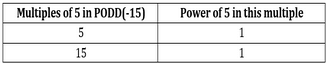• Since the multiples of 5 and 15 occurs twice in PODD(-15), power of 5 in PODD(-15) = (1+1)*2 = 4
• Since the power of 5 in Option II is greater than the power of 5 in PODD(-15), 55 will not divide PODD(-15) completely.
• Finding the power of 7 in PODD(-15) and evaluating Option III
• The only multiple of 7 in PODD(-15) is 7 itself.
• Since 7 occurs twice in PODD(-15), the power of 7 in PODD(-15) = 1*2 = 2
• Since the power of 7 in Option III is greater than the power of 5 in PODD(-15), 73 will not divide PODD(-15) completely.
• Thus we see that only Options II and III will not divide PODD(-15) completely.

Looking at the answer choices, we see that the correct answer is Option E

QUESTION: 19

If x > 0 such that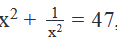what is the value of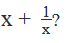Solution:

Given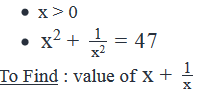Approach

1. We need to find the value of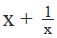and we are given the value of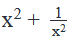1. We can observe from the above 2 expressions thatis of the form a + b andis of the form a2 +b2
2. We also know that (a+b)2=a2+b2+2ab
3. We will use the above relation along with the constraint that x > 0, to find out the value ofWorking Out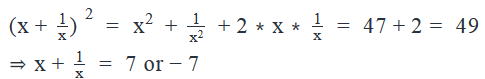As x > 0,cannot be equal to -7. Hence,=7

QUESTION: 20

If x and y are distinct positive integers, such that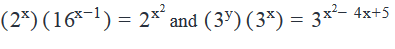What is the value of (x)(y+1) ?

Solution:

Given Info:

• x and y are distinct positive integers. This means x≥1, y≥1 & x≠y
• Also Given are two equations in x and y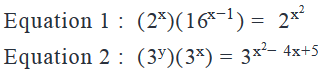To Find:

• Value of (x)(y+1)
• So we need to find the values of x & y in order to find the value of the expression (x)(y+1).

Approach:

• From the 1st equation, which is an equation in x, we will find the values of x.
• We will put the values of x derived from the first equation in equation 2, to find the values of y.
• Knowing the values of x & y and the set of constraints on the value of x & y, we will calculate the value of  (x)(y+1) to arrive at the final answer.

Working out: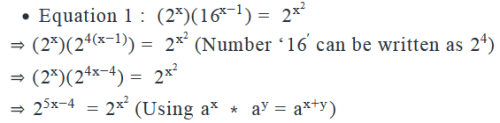On comparing both sides of the above equation as both are to the same base 2, we get

⇒x2=5x−4

Subtracting 5x - 4 from both sides we get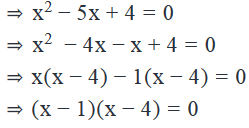Sowehavex=1 or x=4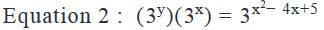• Case 1: x=1 (Value derived from Equation 1)
• Putting x=1 in Equation 2, we get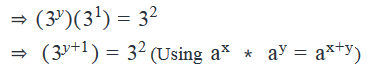• On comparing both sides of the above equation as both are to the same base 3, we get

⇒ y+1=2

⇒ y=1

Case 2: x=4 (Value derived from Equation 1)

• Putting x=4 in Equation 2, we get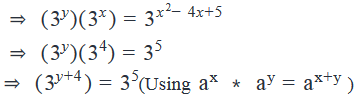• On comparing both sides of the above equation as both are to the same base 3, we get

⇒ y + 4 = 5

⇒ y = 1

• So, we have two pairs of values of x & y

Pair1:(x,y)=(1,1)(FromCase1)

Pair2:(x,y)=(4,1)(FromCase2)

• We have to reject the value, when (x,y) = (1,1), as we have already established above that x≠y.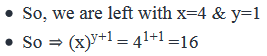Use Code STAYHOME200 and get INR 200 additional OFF Use Coupon Code